# How to use LINEST function in Excel - formula examples

This tutorial explains the syntax of the LINEST function and shows how to use it to do linear regression analysis in Excel.

Microsoft Excel is not a statistical program, however, it does have a number of statistical functions. One of such functions is LINEST, which is designed to perform linear regression analysis and return related statistics. In this tutorial for beginners, we will touch only lightly on theory and underlying calculations. Our main focus will be on providing you with a formula that simply works and can be easily customized for your data.

## Excel LINEST function - syntax and basic uses

The LINEST function calculates the statistics for a straight line that explains the relationship between the independent variable and one or more dependent variables, and returns an array describing the line. The function uses the least squares method to find the best fit for your data. The equation for the line is as follows.

y = bx + a
##### Multiple regression equation:
y = b1x1 + b2x2 + … + bnxn + a

Where:

• y - the dependent variable you are trying to predict.
• x - the independent variable you are using to predict y.
• a - the intercept (indicates where the line intersects the Y axis).
• b - the slope (indicates the steepness of the regression line, i.e. the rate of change for y as x changes).

In its basic form, the LINEST function returns the intercept (a) and the slope (b) for the regression equation. Optionally, it can also return additional statistics for the regression analysis as shown in this example.

### LINEST function syntax

The syntax of the Excel LINEST function is as follows:

LINEST(known_y's, [known_x's], [const], [stats])

Where:

• known_y's  (required) is a range of the dependent y-values in the regression equation. Usually, it is a single column or a single row.
• known_x's  (optional) is a range of the independent x-values. If omitted, it is assumed to be the array {1,2,3,...} of the same size as known_y's.
• const (optional) - a logical value that determines how the intercept (constant a) should be treated:
• If TRUE or omitted, the constant a is calculated normally.
• If FALSE, the constant a is forced to 0 and the slope (b coefficient) is calculated to fit y=bx.
• stats (optional) is a logical value that determines whether to output additional statistics or not:
• If TRUE, the LINEST function returns an array with additional regression statistics.
• If FALSE or omitted, LINEST only returns the intercept constant and slope coefficient(s).
Note. Since LINEST returns an array of values, it must be entered as an array formula by pressing the Ctrl + Shift + Enter shortcut. If it is entered as a regular formula, only the first slope coefficient is returned.

### Additional statistics returned by LINEST

The stats argument set to TRUE instructs the LINEST function to return the following statistics for your regression analysis:

 Statistic Description Slope coefficient b value in y = bx + a Intercept constant a value in y = bx + a Standard error of slope The standard error value(s) for the b coefficient(s). Standard error of intercept The standard error value for the constant a. Coefficient of determination (R2) Indicates how well the regression equation explains the relationship among the variables. Standard error for the Y estimate Shows the precision of the regression analysis. F statistic, or the F-observed value It is used to do the F-test for the null hypothesis to determine the overall goodness of fit of the model. Degrees of freedom (df) The number of degrees of freedom. Regression sum of squares Indicates how much of the variation in the dependent variable is explained by the model. Residual sum of squares Measures the amount of variance in the dependent variable that is not explained by your regression model.

The below map shows the order in which LINEST returns an array of statistics: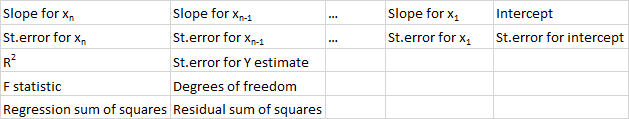In the last three rows, the #N/A errors will appear in the third and subsequent columns that are not filled with data. It is the default behavior of the LINEST function, but if you'd like to hide the error notations, wrap your LINEST formula into IFERROR as shown in this example.

## How to use LINEST in Excel - formula examples

The LINEST function might be tricky to use, especially for novices, because you should not only build a formula correctly, but also properly interpret its output. Below, you will find a few examples of using LINEST formulas in Excel that will hopefully help to sink the theoretical knowledge in :)

### Simple linear regression: calculate slope and intercept

To get the intercept and the slope of a regression line, you use the LINEST function in its simplest form: supply a range of the dependent values for the known_y's argument and a range of the independent values for the known_x's argument. The last two arguments can be set to TRUE or omitted.

For example, with y values (sales numbers) in C2:C13 and x values (advertising cost) in B2:B13, our linear regression formula is as simple as:

`=LINEST(C2:C13,B2:B13)`

To enter it correctly in your worksheet, select two adjacent cells in the same row, E2:F2 in this example, type the formula, and press Ctrl + Shift + Enter to complete it.

The formula will return the slope coefficient in the first cell (E2) and the intercept constant in the second cell (F2):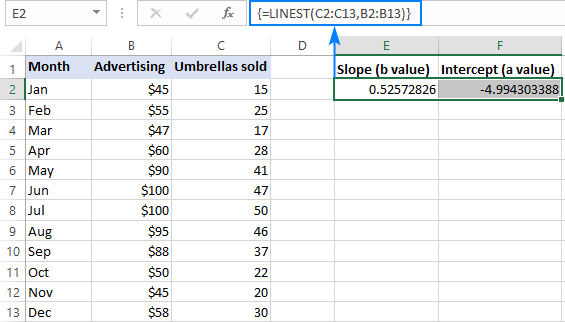The slope is approximately 0.52 (rounded to two decimal places). It means that when x increases by 1, y increases by 0.52.

The Y-intercept is negative -4.99. It is the expected value of y when x=0. If plotted on a graph, it is the value at which the regression line crosses the y-axis.

Supply the above values to a simple linear regression equation, and you will get the following formula to predict the sales number based on the advertising cost:

`y = 0.52*x - 4.99`

For example, if you spend \$50 on advertising, you are expected to sell 21 umbrellas:

`0.52*50 - 4.99 = 21.01`

The slope and intercept values can also be obtained separately by using the corresponding function or by nesting the LINEST formula into INDEX:

Slope

`=SLOPE(C2:C13,B2:B13)`

`=INDEX(LINEST(C2:C13,B2:B13),1)`

Intercept

`=INTERCEPT(C2:C13,B2:B13)`

`=INDEX(LINEST(C2:C13,B2:B13),2)`

As shown in the screenshot below, all three formulas yield the same results: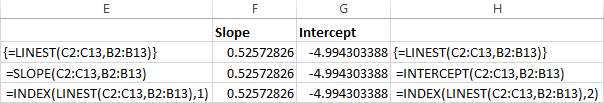### Multiple linear regression: slope and intercept

In case you have two or more independent variables, be sure to input them in adjacent columns, and supply that whole range to the known_x's argument.

For example, with sales numbers (y values) in D2:D13, advertising cost (one set of x values) in B2:B13 and average monthly rainfall (another set of x values) in C2:C13, you use this formula:

`=LINEST(D2:D13,B2:C13)`

As formula is going to return an array of 3 values (2 slope coefficients and the intercept constant), we select three contiguous cells in the same row, enter the formula and press the Ctrl + Shift + Enter shortcut.

Please note that the multiple regression formula returns the slope coefficients in the reverse order of the independent variables (from right to left), that is bn, bn-1, …, b2, b1: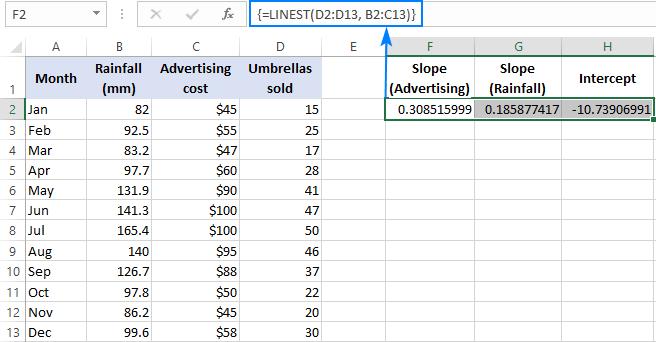To predict the sales number, we supply the values returned by the LINEST formula to the multiple regression equation:

y = 0.3*x2 + 0.19*x1- 10.74

For example, with \$50 spent on advertising and an average monthly rainfall of 100 mm, you are expected to sell approximately 23 umbrellas:

`0.3*50 + 0.19*100 - 10.74 = 23.26`

### Simple linear regression: predict dependent variable

Apart from calculating the a and b values for the regression equation, the Excel LINEST function can also estimate the dependent variable (y) based on the known independent variable (x). For this, you use LINEST in combination with the SUM or SUMPRODUCT function.

For example, here's how you can calculate the number of umbrella sales for the next month, say October, based on sales in the previous months and October's advertising budget of \$50:

`=SUM(LINEST(C2:C10, B2:B10)*{50,1})`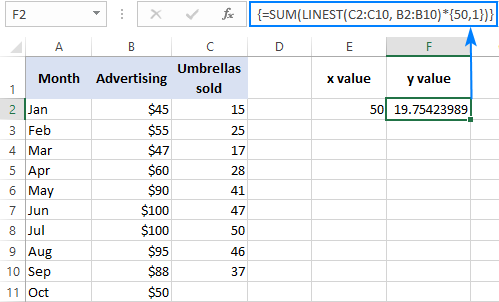Instead of hardcoding the x value in the formula, you can provide it as a cell reference. In this case, you need to input the 1 constant in some cell too because you cannot mix references and values in an array constant.

With the x value in E2 and the constant 1 in F2, either of the below formulas will work a treat:

Regular formula (entered by pressing Enter):

`=SUMPRODUCT(LINEST(C2:C10, B2:B10)*(E2:F2))`

Array formula (entered by pressing Ctrl + Shift + Enter):

`=SUM(LINEST(C2:C10, B2:B10)*(E2:F2))`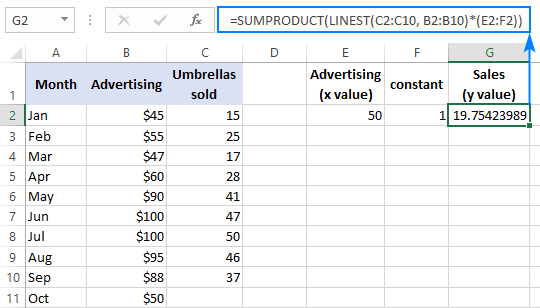To verify the result, you can get the intercept and slope for the same data, and then use the linear regression formula to calculate y:

`=E2*G2+F2`

Where E2 is the slope, G2 is the x value, and F2 is the intercept: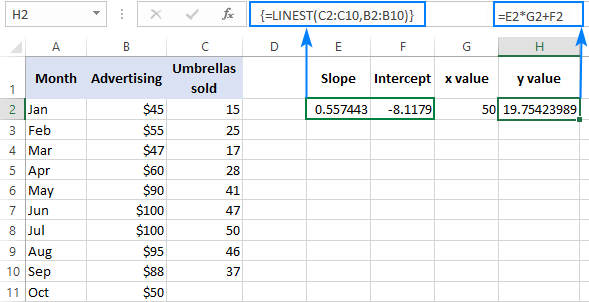### Multiple regression: predict dependent variable

In case you are dealing with several predictors, i.e. a few different sets of x values, include all those predictors in the array constant. For example, with the advertising budget of \$50 (x2) and an average monthly rainfall of 100 mm (x1), the formula goes as follows:

`=SUM(LINEST(D2:D10, B2:C10)*{50,100,1})`

Where D2:D10 are the known y values and B2:C10 are two sets of x values: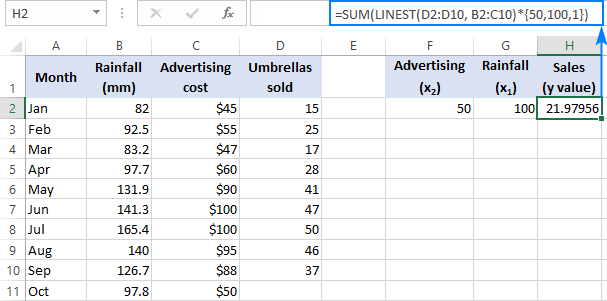Please pay attention to the order of the x values in the array constant. As pointed out earlier, when the Excel LINEST function is used to do multiple regression, it returns the slope coefficients from right to left. In our example, the Advertising coefficient is returned first, and then the Rainfall coefficient. To calculate the predicted sales number correctly, you need to multiply the coefficients by the corresponding x values, so you put the elements of the array constant in this order: {50,100,1}. The last element is 1, because the last value returned by LINEST is the intercept that should not be changed, so you simply multiply it by 1.

Instead of using an array constant, you can input all the x variables in some cells, and reference those cells in your formula like we did in the previous example.

Regular formula:

`=SUMPRODUCT(LINEST(D2:D10, B2:C10)*(F2:H2))`

Array formula:

`=SUM(LINEST(D2:D10, B2:C10)*(F2:H2))`

Where F2 and G2 are the x values and H2 is 1: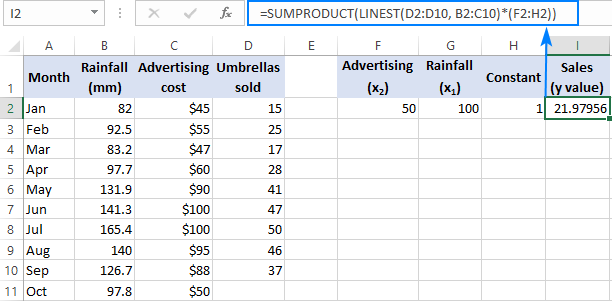### LINEST formula: additional regression statistics

As you may remember, to get more statistics for your regression analysis, you put TRUE in the last argument of the LINEST function. Applied to our sample data, the formula takes the following shape:

`=LINEST(D2:D13, B2:C13, TRUE, TRUE)`

As we have 2 independent variables in columns B and C, we select a rage consisting of 3 rows (two x values + intercept) and 5 columns, enter the above formula, press Ctrl + Shift + Enter, and get this result: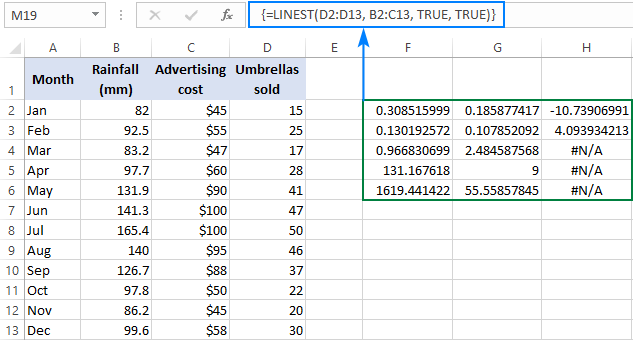To get rid of the #N/A errors, you can nest LINEST into IFERROR like this:

`=IFERROR(LINEST(D2:D13, B2:C13, TRUE, TRUE), "")`

The screenshot below demonstrates the result and explains what each number means: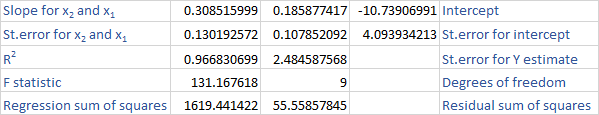The slope coefficients and the Y-intercept were explained in the previous examples, so let's have a quick look at the other statistics.

Coefficient of determination (R2). The value of R2 is the result of dividing the regression sum of squares by the total sum of squares. It tells you how many y values are explained by x variables. It can be any number from 0 to 1, that is 0% to 100%. In this example, R2 is approximately 0.97, meaning that 97% of our dependent variables (umbrella sales) are explained by the independent variables (advertising + average monthly rainfall), which is an excellent fit!

Standard errors. Generally, these values show the precision of the regression analysis. The smaller the numbers, the more certain you can be about your regression model.

F statistic. You use the F statistic to support or reject the null hypothesis. It is recommended to use the F statistic in combination with the P value when deciding if the overall results are significant.

Degrees of freedom (df). The LINEST function in Excel returns the residual degrees of freedom, which is the total df minus the regression df. You can use the degrees of freedom to get F-critical values in a statistical table, and then compare the F-critical values to the F statistic to determine a confidence level for your model.

Regression sum of squares (aka the explained sum of squares, or model sum of squares). It is the sum of the squared differences between the predicted y-values and the mean of y, calculated with this formula: =∑(ŷ - ȳ)2. It indicates how much of the variation in the dependent variable your regression model explains.

Residual sum of squares. It is the sum of the squared differences between the actual y-values and the predicted y-values. It indicates how much of the variation in the dependent variable your model does not explain. The smaller the residual sum of squares compared with the total sum of squares, the better your regression model fits your data.

## 5 things you should know about LINEST function

To efficiently use LINEST formulas in your worksheets, you may want to know a bit more about the "inner mechanics" of the function:

1. Known_y's and known_x's. In a simple linear regression model with only one set of x variables, known_y's and known_x's can be ranges of any shape as long as they have the same number of rows and columns. If you do multiple regression analysis with more than one set of independent x variables, known_y's must be a vector, i.e. a range of one row or one column.
2. Forcing the constant to zero. When the const argument is TRUE or is omitted, the a constant (intercept) is calculated and included in the equation: y=bx + a. If const is set to FALSE, the intercept is considered to be equal 0 and omitted from the regression equation: y=bx.

In statistics, it has been debated for decades whether it makes sense to force the intercept constant to 0 or not. Many credible regression analysis practitioners believe that if setting the intercept to zero (const=FALSE) appears to be useful, then linear regression itself is a wrong model for the data set. Others suppose that the constant can be forced to zero in certain situations, for example, in the context of regression discontinuity designs. In general, it is recommended to go with the default const=TRUE or omitted in most cases.

3. Accuracy. The accuracy of the regression equation calculated by the LINEST function depends on the dispersion of your data points. The more linear the data, the more accurate the results of the LINEST formula.
4. Redundant x values. In some situations, one or more independent x variables might have no additional predictive value, and removing such variables from the regression model does not affect the accuracy of the predicted y values. This phenomenon is known as "collinearity". The Excel LINEST function checks for collinearity and omits any redundant x variables that it identifies from the model. The omitted x variables can be recognized by 0 coefficients and 0 standard error values.
5. LINEST vs. SLOPE and INTERCEPT. The underlying algorithmic of the LINEST function differs from the algorithm used in the SLOPE and INTERCEPT functions. Therefore, when the source data is undetermined or collinear, these functions may return different results.

## Excel LINEST function not working

If your LINEST formula throws an error or produces a wrong output, chances are it's because of one of the following reasons:

1. If the LINEST function returns just one number (slope coefficient), most likely you have entered it as a regular formula, not an array formula. Be sure to press Ctrl + Shift + Enter to complete the formula correctly. When you do this, the formula gets enclosed in the {curly brackets} that are visible in the formula bar.
2. #REF! error. Occurs if the known_x's and known_y's ranges have different dimensions.
3. #VALUE! error. Occurs if known_x's or known_y's contains at least one blank cell, text value or text representation of a number that Excel does not recognize as a numeric value. Also, the #VALUE error occurs if the const or stats argument cannot be evaluated to TRUE or FALSE.

That's how you use LINEST in Excel for a simple and multiple linear regression analysis. To have a closer look the formulas discussed in this tutorial, you are welcome to download our sample Excel LINEST function workbook. I thank you for reading and hope to see you on our blog next week!

## You may also be interested in

### 15 responses to "How to use LINEST function in Excel - formula examples"

1.Shankar Iyer says:

Dear Ms. Svetlana,
Can you please extend me the kink to the data set of the above example of "Rainfall-Advertising-Umbrella" example for multiple regression. Do you have other new data sets on which I can work on independently and he respective solutions for the same.?
Thanks
Shankar

•Svetlana Cheusheva (Ablebits.com Team) says:

Dear Shankar,

2.chris says:

You might be the best in Excel. I don't know whether any published books or existing pages are better than this, not only comprehensive but deep detail. I happened to visit your site while I am drafting my web page for a program (finding the best fit regression equation out of 9 equations) and to display the trend line of best fit on X-Y chart) in order to explain Linest function and Index function referring to Linest function's result matrix. If you have any interest in my program, I may send you the program with draft page.
Cheers
Chris.

•Svetlana Cheusheva (Ablebits.com Team) says:

Hi Chis,

Sorry for my delayed response, it looks like our blog engine did not notify me about your comment in a timely manner. Thank you so much for your kind words and proposal! I guess it's too late now, just wanted to say thanks anyway :)

3.tony says:

Control Shift enter (guess you mean return) doesn't work.

•Staurt says:

Hi Tony.
In my experience following these instructions, the sequence is very important, i.e.
Select the output cells.
Type the formula in the formula bar.
Press Cntrl+Shift+Enter (on my windows keyboard).
At least it worked for me.

•Stuart says:

and as you can see from the fact that I mis-spelled my own name, my keyboard skills are not the best :)

•Svetlana Cheusheva (Ablebits.com Team) says:

Hi Stuart,

Thank you for your support! I always proofread my posts twice but those annoying typos still manage to sneak in every now and then :)

•Svetlana Cheusheva (Ablebits.com Team) says:

Hi Tony,

Array formulas are always entered via "Control + Shift + Enter" in Excel, therefore they are sometimes called CSE formulas. (I am talking about Excel for Windows, didn't have a chance to check this in Excel for Mac.) You can find more information in How to enter array formula in Excel.

You can also download our sample workbook to see all the formulas in action.

4.Stuart says:

Excellent post as usual.
Thanks Svetlana.

5.Ershad says:

Hello,
Could you give me the exact formula of linest? Actually, I want to implement it in Matlab, and I do not know how exactly the linest working.

Thank you

6.Hebe says:

Hi, can you use LINEST to calculate a multivariate regression where several of the variables are dummy variables (i.e for gender male=1,female=0)
Thanks

7.UMAPATHI says:

Hello,
How to find regression coefficient values from system of multiple equation in excel 2007 for 5X5 matrix
V1 = a11V1+a12V2+a13V3+a14V4+a15V5
V2 = a21V1+a22V2+a23V3+24V4+a25V5
V3 = a31V1+a32V2+a33V3+34V4+a35V5
V4 = a41V1+a42V2+a43V3+44V4+a45V5
V5 = a51V1+a52V2+a53V3+54V4+a55V5
here V1,V2, V3, V4 and V5 values are known and N = total number, by using these we need to find coefficient values.

8.Abhi says:

Hi
Your example saved my life, Never knew Excel is so powerful.
Thanks

9.Mahesh says:

How to use excel formula for standard addition method to calculate negative intercept on x axis